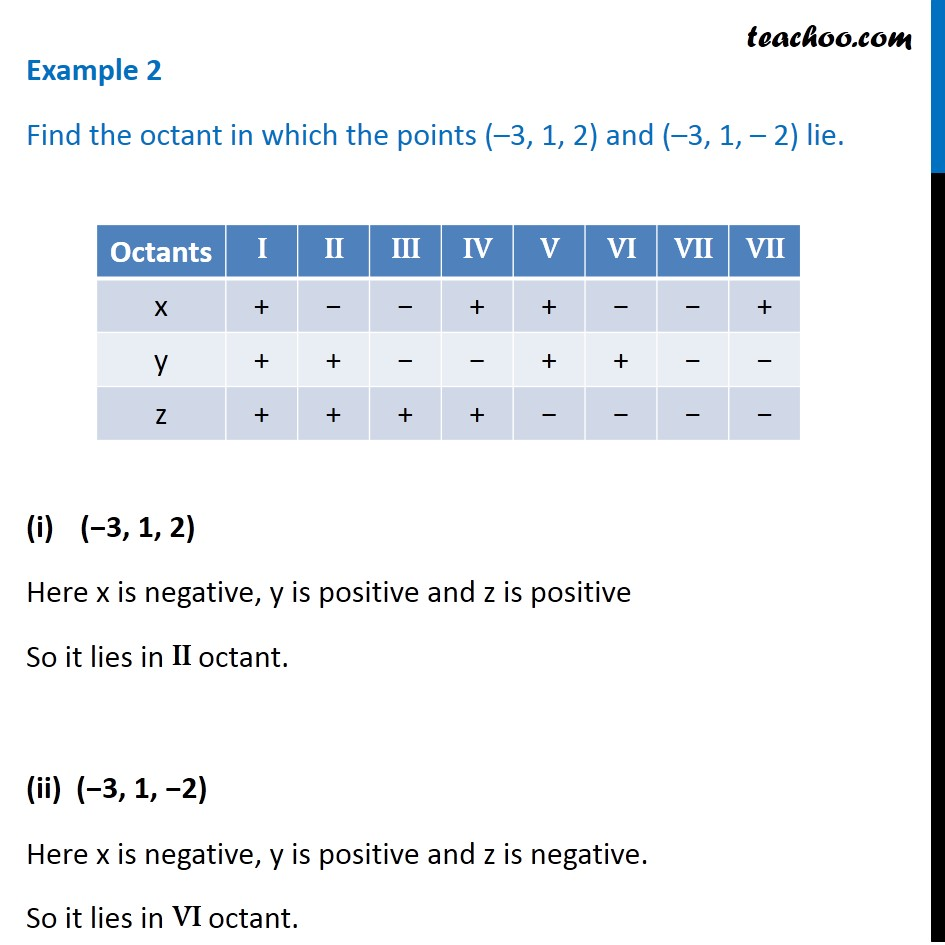Examples

Chapter 12 Class 11 - Intro to Three Dimensional Geometry (Term 2)
Serial order wise983 students joined Teachoo Black. What are you waiting for?

### Transcript

Example 2 Find the octant in which the points (–3, 1, 2) and (–3, 1, – 2) lie. (−3, 1, 2) Here x is negative, y is positive and z is positive So it lies in II octant. (ii) (−3, 1, −2) Here x is negative, y is positive and z is negative. So it lies in VI octant.